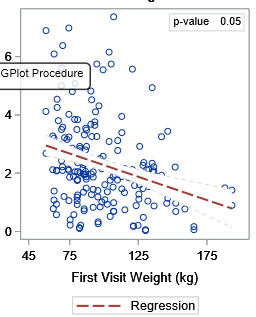## Plotting 95% CI with SGPLOT

I was trying to use this code as reference: https://communities.sas.com/t5/Graphics-Programming/How-Can-I-Display-Confidence-Interval-like-Predi...

However, the 95% CI of my graph display overlaps the data points. I want either the background color of the 95% CI very faded or nonexistent.

``````%macro Graphics2(tissue=, units=, DV=, adjust=, pval=);
title; title1; footnote1; footnote2;
ods graphics / reset width = 300px height = 400px noscale attrpriority = none;

proc sgplot data = work (where = (SPECTYPE = "&tissue"));
scatter x = V1WT y = &DV;
reg x = V1WT y = &DV / name = "reg" clm clmattrs=(clmfillattrs=(color=white) clmlineattrs=(pattern=shortdash)) nolegclm
nomarkers lineattrs = GraphData2; /*add regression line*/
keylegend "reg";
xaxis label = "Weight (kg)" values = (45, 50 to 200 by 25);
%if &adjust = Y %then %do;
yaxis label = "Concentrations (&units)";
title height = 10pt "Concentrations vs. Weight";
footnote1 j = l height = 8pt "Note: Concentrations were adjusted for dose received at time of sample taken";
%end;
%else %if &adjust = N %then %do;
yaxis label = "Concentrations (&units)";
title height = 10pt "&tissue: Concentrations vs. Weight";
%end;
inset ("p-value" = "&pval") / border textattrs = (size=8);
run;
%mend;

``````1 ACCEPTED SOLUTION

Accepted Solutions

## Re: Plotting 95% CI with SGPLOT

@mariko5797 wrote:

I was trying to use this code as reference: https://communities.sas.com/t5/Graphics-Programming/How-Can-I-Display-Confidence-Interval-like-Predi...

However, the 95% CI of my graph display overlaps the data points. I want either the background color of the 95% CI very faded or nonexistent.

``````%macro Graphics2(tissue=, units=, DV=, adjust=, pval=);
title; title1; footnote1; footnote2;
ods graphics / reset width = 300px height = 400px noscale attrpriority = none;

proc sgplot data = work (where = (SPECTYPE = "&tissue"));
scatter x = V1WT y = &DV;
reg x = V1WT y = &DV / name = "reg" clm clmattrs=(clmfillattrs=(color=white) clmlineattrs=(pattern=shortdash)) nolegclm
nomarkers lineattrs = GraphData2; /*add regression line*/
keylegend "reg";
xaxis label = "Weight (kg)" values = (45, 50 to 200 by 25);
%if &adjust = Y %then %do;
yaxis label = "Concentrations (&units)";
title height = 10pt "Concentrations vs. Weight";
footnote1 j = l height = 8pt "Note: Concentrations were adjusted for dose received at time of sample taken";
%end;
%else %if &adjust = N %then %do;
yaxis label = "Concentrations (&units)";
title height = 10pt "&tissue: Concentrations vs. Weight";
%end;
inset ("p-value" = "&pval") / border textattrs = (size=8);
run;
%mend;

Easiest thing to do would be the change the order of the Scatter and Reg plots. If you place the Scatter after the Reg it will be placed after (or over if you want to think of it that way) the regression.

Second would be to use the CLMTRANSPARENCY option with a value of 0.5 or greater (up to 1 for completely transparent). The default is 0 for this parameter which makes the confidence band opaque.

## Re: Plotting 95% CI with SGPLOT

@mariko5797 wrote:

I was trying to use this code as reference: https://communities.sas.com/t5/Graphics-Programming/How-Can-I-Display-Confidence-Interval-like-Predi...

However, the 95% CI of my graph display overlaps the data points. I want either the background color of the 95% CI very faded or nonexistent.

``````%macro Graphics2(tissue=, units=, DV=, adjust=, pval=);
title; title1; footnote1; footnote2;
ods graphics / reset width = 300px height = 400px noscale attrpriority = none;

proc sgplot data = work (where = (SPECTYPE = "&tissue"));
scatter x = V1WT y = &DV;
reg x = V1WT y = &DV / name = "reg" clm clmattrs=(clmfillattrs=(color=white) clmlineattrs=(pattern=shortdash)) nolegclm
nomarkers lineattrs = GraphData2; /*add regression line*/
keylegend "reg";
xaxis label = "Weight (kg)" values = (45, 50 to 200 by 25);
%if &adjust = Y %then %do;
yaxis label = "Concentrations (&units)";
title height = 10pt "Concentrations vs. Weight";
footnote1 j = l height = 8pt "Note: Concentrations were adjusted for dose received at time of sample taken";
%end;
%else %if &adjust = N %then %do;
yaxis label = "Concentrations (&units)";
title height = 10pt "&tissue: Concentrations vs. Weight";
%end;
inset ("p-value" = "&pval") / border textattrs = (size=8);
run;
%mend;